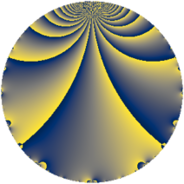# Properties

 Label 1089.3.bLevel $1089$ Weight $3$ Character orbit 1089.b Rep. character $\chi_{1089}(485,\cdot)$ Character field $\Q$ Dimension $72$ Newform subspaces $10$ Sturm bound $396$ Trace bound $7$

# Related objects

## Defining parameters

 Level: $$N$$ $$=$$ $$1089 = 3^{2} \cdot 11^{2}$$ Weight: $$k$$ $$=$$ $$3$$ Character orbit: $$[\chi]$$ $$=$$ 1089.b (of order $$2$$ and degree $$1$$) Character conductor: $$\operatorname{cond}(\chi)$$ $$=$$ $$3$$ Character field: $$\Q$$ Newform subspaces: $$10$$ Sturm bound: $$396$$ Trace bound: $$7$$ Distinguishing $$T_p$$: $$2$$, $$7$$

## Dimensions

The following table gives the dimensions of various subspaces of $$M_{3}(1089, [\chi])$$.

Total New Old
Modular forms 288 72 216
Cusp forms 240 72 168
Eisenstein series 48 0 48

## Trace form

 $$72q - 144q^{4} - 16q^{7} + O(q^{10})$$ $$72q - 144q^{4} - 16q^{7} + 48q^{10} + 8q^{13} + 216q^{16} - 40q^{19} - 288q^{25} + 32q^{28} + 56q^{31} + 200q^{34} - 104q^{37} - 432q^{40} + 104q^{43} - 24q^{46} + 592q^{49} - 280q^{52} + 536q^{58} + 8q^{61} - 168q^{64} - 144q^{67} + 64q^{70} - 448q^{73} + 344q^{76} - 448q^{79} - 744q^{82} - 48q^{85} + 632q^{91} - 360q^{94} + 640q^{97} + O(q^{100})$$

## Decomposition of $$S_{3}^{\mathrm{new}}(1089, [\chi])$$ into newform subspaces

Label Dim. $$A$$ Field CM Traces $q$-expansion
$$a_2$$ $$a_3$$ $$a_5$$ $$a_7$$
1089.3.b.a $$2$$ $$29.673$$ $$\Q(\sqrt{-2})$$ None $$0$$ $$0$$ $$0$$ $$-2$$ $$q+\beta q^{2}+2q^{4}-4\beta q^{5}-q^{7}+6\beta q^{8}+\cdots$$
1089.3.b.b $$2$$ $$29.673$$ $$\Q(\sqrt{-2})$$ None $$0$$ $$0$$ $$0$$ $$2$$ $$q+\beta q^{2}+2q^{4}+4\beta q^{5}+q^{7}+6\beta q^{8}+\cdots$$
1089.3.b.c $$4$$ $$29.673$$ $$\Q(\sqrt{-2}, \sqrt{15})$$ None $$0$$ $$0$$ $$0$$ $$-20$$ $$q+\beta _{1}q^{2}+(-4+\beta _{3})q^{4}+(-\beta _{1}+2\beta _{2}+\cdots)q^{5}+\cdots$$
1089.3.b.d $$4$$ $$29.673$$ $$\Q(\sqrt{-2}, \sqrt{15})$$ None $$0$$ $$0$$ $$0$$ $$20$$ $$q+\beta _{1}q^{2}+(-4+\beta _{3})q^{4}+(\beta _{1}-2\beta _{2}+\cdots)q^{5}+\cdots$$
1089.3.b.e $$4$$ $$29.673$$ $$\Q(\sqrt{-2}, \sqrt{3})$$ None $$0$$ $$0$$ $$0$$ $$0$$ $$q-\beta _{1}q^{2}-2q^{4}+2\beta _{3}q^{5}-7\beta _{2}q^{7}+\cdots$$
1089.3.b.f $$8$$ $$29.673$$ 8.0.$$\cdots$$.10 None $$0$$ $$0$$ $$0$$ $$0$$ $$q+\beta _{1}q^{2}+(-4-\beta _{2})q^{4}+(-\beta _{6}-\beta _{7})q^{5}+\cdots$$
1089.3.b.g $$8$$ $$29.673$$ 8.0.$$\cdots$$.6 None $$0$$ $$0$$ $$0$$ $$-16$$ $$q-\beta _{2}q^{2}+(-2-\beta _{3})q^{4}+(-\beta _{1}+\beta _{2}+\cdots)q^{5}+\cdots$$
1089.3.b.h $$8$$ $$29.673$$ 8.0.3317760000.7 None $$0$$ $$0$$ $$0$$ $$0$$ $$q-\beta _{7}q^{2}+\beta _{3}q^{4}-\beta _{1}q^{5}+(\beta _{2}+\beta _{4}+\cdots)q^{7}+\cdots$$
1089.3.b.i $$16$$ $$29.673$$ $$\mathbb{Q}[x]/(x^{16} + \cdots)$$ None $$0$$ $$0$$ $$0$$ $$-8$$ $$q+\beta _{1}q^{2}+(-2+\beta _{2})q^{4}+\beta _{13}q^{5}+\cdots$$
1089.3.b.j $$16$$ $$29.673$$ $$\mathbb{Q}[x]/(x^{16} + \cdots)$$ None $$0$$ $$0$$ $$0$$ $$8$$ $$q+\beta _{1}q^{2}+(-2+\beta _{2})q^{4}-\beta _{13}q^{5}+\cdots$$

## Decomposition of $$S_{3}^{\mathrm{old}}(1089, [\chi])$$ into lower level spaces

$$S_{3}^{\mathrm{old}}(1089, [\chi]) \cong$$ $$S_{3}^{\mathrm{new}}(33, [\chi])$$$$^{\oplus 4}$$$$\oplus$$$$S_{3}^{\mathrm{new}}(99, [\chi])$$$$^{\oplus 2}$$$$\oplus$$$$S_{3}^{\mathrm{new}}(363, [\chi])$$$$^{\oplus 2}$$